# 2nd PUC Basic Maths Question Bank Chapter 10 Learning Curve

Students can Download Basic Maths Question Bank Chapter 10 Learning Curve Questions and Answers, Notes Pdf, 2nd PUC Basic Maths Question Bank with Answers helps you to revise the complete Karnataka State Board Syllabus and to clear all their doubts, score well in final exams.

## Karnataka 2nd PUC Basic Maths Question Bank Chapter 10 Learning Curve

### 2nd PUC Basic Maths Learning Curve One Mark Questions and Answers

Question 1.
Find the index of learning for 70% learning effect
Index of learning for 70%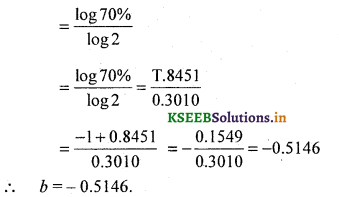Question 2.
What do you mean by 80% learning effect?
80% learning effect means that when the cumulative output is doubled the cumulative average labour hours per unit will be 80% of the previous level.

Question 3.
Find the index of learning for 80% learning effect.
b = Index of learningQuestion 4.
The ave labour cost for first 100 units produced by a firm is Rs. 25. When the output was double to 200 units, the ave labour cost was Rs. 20. Determine learning curve ratio and learning curve percent.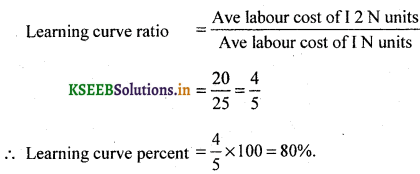Question 5.
The ave labour cost of a production for 100 units is Rs. 75. If the learning curve ratio is 0.75. Find the ave cost of production for 50 units.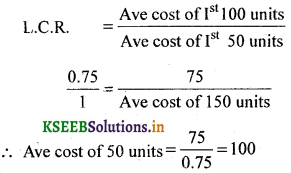Question 6.
The ave labour cost of 100 units is Rs. 0.5 The learning curve ratio is 0.5. What is the ave labour cost of 200 units.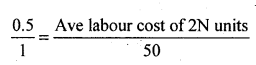Ave labour cost of 2N units= 0.5 x 50 = Rs. 25.Question 7.
The ave labour cost of 1st 150 units of production by a factory is Rs. 100. When the out put was doubled to 300 units, the ave labour cost was Rs. 50. Find the L.C.R. and the reaming curve percent.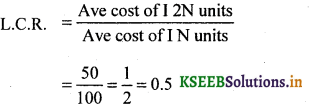Learning curve percent = 0.5 x 100 = 50%.

Question 8.
Calculate the index of learning for 90% learning effect.### 2nd PUC Basic Maths Learning Curve Five Marks Questions and Answers

Question 9.
The time required to produce the first unit of a product is 1000 hrs. If the manufactures experiences 80% learning effect calculate the are time per unit and the time taken to produce altogether 8 units. Also find the total labour charges for the production of 8 units at the rate of Rs. 12.50 per hour.
I Table Method

 Units produced Total out put Cumulative ave time percent Total no. of hours 1 1 1000 1000 1 2 80% of 1000 = 800 800 x 2 = 1600 2 4 80% of 800 = 640 640 x 4 = 2560 4 8 80% of 640 = 512 512 x 8 = 4096

Total time to produce 8 units = 4096 Hrs.
Given Labour charges &r 1 hour = Rs. 12.50
∴ Labour charges for 4096 hours = 4096 x 12.50
= 51,200II Formula method:-

Given a = 1000, x = 8 units.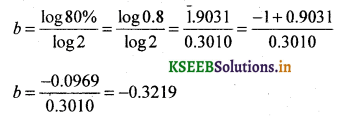0.3010
Y = axh
log Y = log a + b log X
= log 1000 + (-0.3219) log 8         .
= 3.000 -0.3219 x 0.9031
= 3.000-0.2907 log Y
= 2.7093
Y =A.L (2.7093)                                                                                      .
= 512 hrs. per unit
∴ Total time for 8 units = 512 x 8 = 4096 hrs
Labour charges per hour = Rs. 12.50
Labour charges for 4096 hrs = 4096 x 12.50 = 51,200.

Question 10.
An engineering company has won the contract for supplying aircraft engines of a new type. The prototype constructed to win the contract took 500 hrs. it is expected that there will be 90% learning effect. Estimate the labour cost of producing 8 engines of new order. If the labour cost is Rs. 40 per hour.
Table Method

 Units Produced Total Units(output) Comulativeave time/unit Total hours 1 1 500 500 1 2 90% of 500 = 450 450 x 2 = 900 2 4 90% of 450 = 405 405 x 4 = 1620 4 8 90% of 405 = 364.5 364.5 x 8 = 2916

Total time to produce 8 units = 2916
Given Labour charges per hour = Rs. 40
Labour charges for 2916 hrs = 2916 x 40
= Rs. 1,16,640Formula Method:Y =axh
log Y = log a + b log X
= log 500-0.1521 log 8
= 2.6990-0.1521 x 0.9031
= 2.6990-0.1374
Y =A.L (2.5616)
= 364.43
∴ Ave time / units = 364.43.
Total time to produce 8 units = 364.43 x 8 = 2915.452 = 2916.
Given labour charges / 1hr = Rs. 40 /.
Labour charges for 2916 hrs = 2916 x 40 = 1,16,640.

Question 11.
The manager of a company obtained the following equation for the learning effect
Y = 1450 X-0.2512. The function is based for assembling the first 50 units of the product. Find the Total labour hours required to assemble 100 units.
Given the total time for producing a lot of 50 units of a product.
∴ 100 units would constitue 2 lots of 50 units each.
a = 1450, X = 2, b = -0.2512
Y = a xb
log Y = log a + b log X
= log 1450 – 0.2512 log 2
= 3.1614 – 0.2512 x 0.3010
= 3.1614-0.0756
= 3.0858
Y = A.L (3.0858)= 1216 Hrs per lot
Total time required for 2 lots = 2 x 1216 = 2432 Hrs.

Question 12.
A manager of a company obtained the following equation for the learning effect
y = 200. X-03219. The first unit of the product requires 200 labour hours. If the company is plan­ning to assemble 100 units of the product find the total labour hours required.
Given Y = 200 X-63219 Where X = 100 units
= 200 x (100)-03219
log Y = log 200 – 0.3219 log 100
= 2.3010-0.3219 x 2.0
= 2.3010-0.6438
= 1.6572
Y = A.L. (1.6572)
= 45.41 per unit
∴ Total labour hours for 100 units
= 45.41 x 100 = 4541 hrs.Question 13.
The production manager of an electronic company obtained the following equation for the learning effect Y = 1356 X-0.3219. The function is based on the company’s experience for assembling the first 50 units of the product the company was asked to bid a new order of 100 additional units. Find the labour hours required to assemble 100 units.
Given Y = 1356 X-03219
Let 50 units = 1 lot
= 1356 (2)-03219
∴ 100 units = 2 lots = X
log Y = log 1356 – 0.3219 log 2
= 3.1322 – 0.3219(0.3010)
= 3.1322 – 0.0969
= 3.0353
= A.L (3.0353) = 1085.00 hours/lot
∴ The total no. of labour hours required to assemble 2 lots (100 units)
= 1085 x 2 = 2170 hrs.

Question 14.
An aircraft manufacturer supplies aircraft engine to different Airlines. They have just completed an initial trial order for 30 engines involving a total of 6000 direct labour hours at Rs. 20 per hour. They have been asked to bid for a prospective contract for supply of 90 engines. It is expected that there will be 80% learning effect. Estimate the labour cost for the new order.
Given a = 6000 hrs, 1 lot = 30 engines, X = 120 engines = 4 lots
Tables Method :

 Lots produced Cumulative Total lots Cumulative time Per lot Total hours 1 1 6000 6000 1 2 80% of 6000 = 4800 4800 x 2 = 9600 2 4 80% of 4800 = 3840 3840 x 4 = 15360

Total time taken to produce 120 Engines = 15360
Time taken to produce 30 Engines = 6000
Total time taken to produce 90 Engines = 15360 – 6000 = 9360
Labour cost at Rs. 20 per hour = 9360 x 20 = 1,87,200

Formula Method:
a =6000 hours, X =4.Y = axb
log Y = log a + b log X
= log 6000 – 0.3219 log 4
= 3.7782 – 0.3219 x 0.6021
= 3.7782 – 0.1938
= 3.5844
Y = A.L (3.5844) = 3841
Total no of hours required to produce 4 lots (120 Engines)
= 3841 x 4 = 15364 hours
Total no of hrs required to produce 90 Engines
= 15364-6000 = 9364 hrs.
Labour cost at Rs. 20 per hour
= 20 x 9364 = Rs. 1,87,280.Question 15.
A company requires 253.5 hrs to produce the 1st 30 units. If the learning effect is 80% find the no of hours required to produce an additional no of 120 units. Also find the total labour cost at Rs. 20 / hr.
1 lot = 30 units
120 units = 4 lots

 Lots Produced Total output Camulative ave time per lot (hrs) Total hours 1 1 253.5 253.3 1 2 80% of 253.5 = 202.8 405.6 2 4 80% of 202.8 = 162.24 648.96

Total time required for 120 units = 648.96hrs
Additional time taken for 30 units = 253.5 hrs                                    ‘
∴ Total time required to complete 150 units
= 648.96 + 253.5
= 902.46
Total labour cost at 20 rs / hour
= 902.46 x 20
= 18049.2.

Question 16.
A company requires 2134.2 hours to produce the first 40 machines. If the learning Ef­fect is 80%. Find the number of hours required to produce next 120 machines.
Let 40 units = 1 lot
120 units = 3 lots

 Lots Produced Total out put in lots Cumulative ave time per lot in (hrs) Total hours 1 1 2134.2 2134.2 1 2 80% of 2134.2 = 1707.36 3414.72 2 4 80% of 1707.36 = 1365.88 5463.55

Total time required for 4 lots (160 mechines) = 5463.55
∴ Total time for 3 lots (120 mechines)
= 5463.55 – 2134.20
= 3329.35.Question 17.
The first unit of a product took 80 hours to manufacture. If the worker’s show 80% learning effect. Find the total time taken to produce 6th, 7th and 8th units.

 Units Produced Total output Cumulative ave time per lot Total time 1 1 80 80 1 2 80% of 80 = 64 128 2 4 80% of 64 = 51.2 204.8 4 8 80% of 51.2 = 40.96 327.68

Total time taken to produce 5 units = Time taken to produce 4 units + 1 unit
= 204.8 + 80 = 284.8
∴ The total time taken to produce 6th, 7th and 8th units.
= Time taken to produce 8 units – Time taken to produce 5 units.
= 327.68 – 284.8 = 42.88.

Question 18.
A company requires 100 hrs to produce the 1st ten units at Rs. 15/ per hr. If the learning effect is 80%. Find the total number of hours required to produce 160 units and also the total cost to produce 160 units.
Given:-a = 100 Hrs, 10 Units =1 lot
∴ 160 units = 16 lots
b = index of learning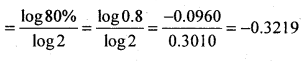log Y = log a + b log X
= log 100 – 0.3219 log 16
= 2 – 0.3219 x 1.2041
= 2 – 0.3875
= 1.6125
Y = A(1.6125) = 40.98 hours/unit.
∴ Total time required for 160 units of 16 lots
= 40.98 x 16
= 655.68 hrs
Total cost to produce 16 units at Rs. 15/hr
= 655.68 x 15
= 9835.20

Table Method :

 Lots produced Total lots Cumulative out put per lot in hrs Total time 1 1 100 100 1 2 80% of 100 = 80 160 2 4 80% of 80 = 64 256 4 8 80% of 64 = 51.2 409.6 8 16 80% of 51.2 = 40.96 655.36

Total time for 16 lots = 655.36 hrs /
Total cost to produce 16 lots at Rs. 15 / hr
= 655.36 x 16
= 9830.40 Rs.Question 19.
ABC company Ltd, has observed that a 90% learning effect applies to all labour related costs whenever a new product is taken up for production. The anticipated production is 320 units for the coming year. The production is done in lots of 10 units each and each lot requires 1000 labour hours at Rs. 16 per hour. You are required to compute the total labour hours and labour costs to manufacture 320 units.
10 units =1 lot
320 units = 32 lots.

 Lots produced Total out put in lots Cumulative average time per lot in hours Total hours 1 1 1000 1000 1 2 90% of 1000 = 900 1800 2 4 90% of 900 = 810 3240 4 8 90% of 810 = 729 5832 8 16 90% of 729 = 656.1 10497.6 16 32 90% of 656.1 = 590.5 18,895.9

Total labour hours for 32 lots = 18, 895.9 hours
Labour cost at 16 Rs. per hour = 18,895.9 x 16
= Rs. 3,02,334.

Question 20.
A company requires 200 hrs to produce the first ten units at Rs. 10 per hour. If the learning effect is Expected is 90%. Find the total no. of hours required to produce 320 units and also the total cost to produce 320 units.